# X-ray crystallography

### X-ray crystallography

A method for determining the arrangement of atoms within a crystal is X-ray crystallography. X-ray diffraction, a non-destructive technique, is based on this technique. When an X-ray beam hits a crystal, the beam can be diffracted. A three-dimensional image of the density of electrons within the crystal may be derived from the angles and intensities of these diffracted beams. The mean positions of the atoms in the crystal can be determined from this electron density. This technique acts as an atomic microscope, to determine the three-dimensional structure of crystals using X-rays instead of visible light. In chemistry and biology, X-ray crystallography has had an enormous impact.

It scatters the incident beam uniformly in all directions when X-rays interact with a single particle. However, the scattered beams can join together in a few directions when X-rays interact with a solid material and strengthen each other to yield diffraction. For the diffraction of the beams, the regularity of the material is responsible. Hence, the molecule must be crystallised for X-ray crystallography. Of many billions of small identical units called unit cells, a crystal is built up. The unit cell is the smallest and simplest element of volume that is fully representative of the crystal as a whole. If the crystal ‘s internal order is poor, then high angles or high resolution will not be diffracted from the X-rays, and the data will not produce a detailed structure. If the crystal is well ordered, diffraction at high angles or high resolution will be measurable; it should result in a detailed structure.

Drawing an analogy with visible light is a good way of understanding X-ray diffraction. Light has certain characteristics that are best defined by considering the nature of waves. Whenever wave phenomena take place in nature, there can be interaction between waves. When waves are in the same phase with each other from two sources, their total amplitude is additive (constructive interference); and if they are out of phase, their amplitude (destructive interference) is reduced. When light passes through two pinholes in a piece of opaque material and then falls to a white surface, this effect can be seen in the figure. With dark regions where light waves are out of phase and bright regions where they are in phase, interference patterns result. If the wavelength of the light (λ) is known, the angle alpha between the original beam and the first diffraction peak can be measured and then the distance d between the two holes can be calculated with the formula.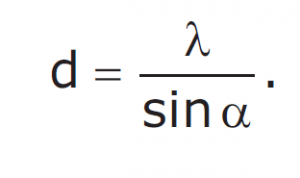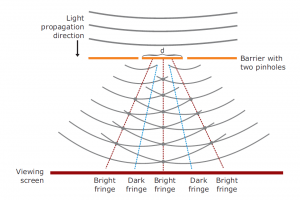Diffraction patterns. Any energy in the form of waves will produce interference patterns if the waves from two or more sources are superimposed in space. One of the simplest patterns can be seen when monochromatic light passes through two neighbouring pinholes and is allowed to fall on a screen. When the light passes through the two pinholes, the holes act as light sources, with waves radiating from each and falling on a white surface. Where the waves are in the same phase, a bright fringe appears (constructive interference), but where the waves are out-of-phase they cancel each other out, producing dark fringe (destructive interference).

To calculate the distance between atoms in crystals, the same approach can be used. We can use a beam of Xrays instead of visible light, which has longer wavelengths to interact with atoms. X-rays are a type of electromagnetic radiation, like light, but they have a much smaller wavelength. The X-ray wavelengths (typically around 0.1 nm) are of the same order of magnitude as the distances in a molecule or crystal between atoms or ions. Most of the X-rays will pass straight through it if a narrow beam of X-rays is directed at a crystalline solid. However, a small fraction disperses, especially with the electrons, by the atoms in the crystal. If they are in phase there, the scattered waves strengthen each other on the film or detector; and if they are out of phase, they cancel each other. Only the atomic arrangement depends on the way in which the scattered waves recombine.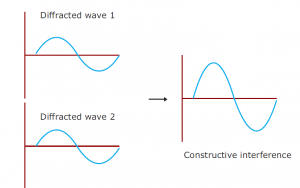Refraction refers to a change in the direction of a source of light, when the light moves from one medium to another while diffraction is a phenomenon that is often described as ‘waves bending around corners’. Diffraction occurs when waves encounter an obstacle.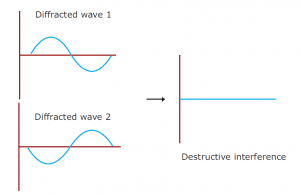Interference occurs among the waves scattered by the atoms when crystalline solids are exposed to X-rays. There are two types of interferences depending on how the waves overlap one another. Constructive interference occurs when the waves are moving in phase with each other; and destructive interference occurs when the waves are out-of-phase.

X-rays are 0.1–100 Å wavelengths of electromagnetic radiation. By bombarding a metal target (most commonly copper, molybdenum or chromium) with electrons generated by a heated filament and accelerated by an electric field, X-rays can be created. A high-energy electron in a target metal atom collides with and displaces an electron from a lower orbital. Then an electron drops into the resulting vacancy from a higher orbital, emitting its excess energy as an X-ray photon. A crystal is placed between the X-ray source and a detector in an X-ray beam, and it generates a regular array of spots called reflections. The spots are created by the diffracted X-ray beam; each atom contributes to each spot in a molecule. The electrons surrounding the atoms are the entities that, not the atomic nuclei, physically interact with the incoming X-ray to diffract them. On a photographic plate, the diffraction pattern is recorded and then analysed to disclose the essence of that lattice.

In the X-ray diffraction pattern, the position and intensity of each spot contains information about the positions of the atoms in the crystal that gave rise to them. An optical scanner accurately measures the position and intensity of each reflection and transmits that information to a computer for analysis in digital form. The position of a reflection can be used to obtain the direction in which the crystal diffracts that particular beam. By measuring the optical absorption of the spot on the film, the intensity of a reflection is obtained, giving a measure of the force of the diffracted beam that created the spot.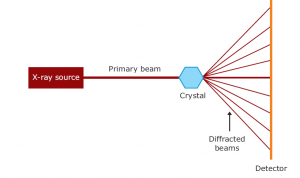In diffraction experiments, a narrow beam of X-ray is taken out from the X-ray source and directed onto the crystal to produce diffracted patterns. When the primary beam hits the crystal, most of it passes straight through, but some is diffracted by the crystal. These diffracted beams are recorded on a detector.

The mathematical expression that describes the interactions of X-rays with crystalline solids is Bragg’s Law. This law describes the relationship between the angle at which a beam of X-rays

REFERENCES